Author: Oscar Cronquist Article last updated on September 30, 2022

###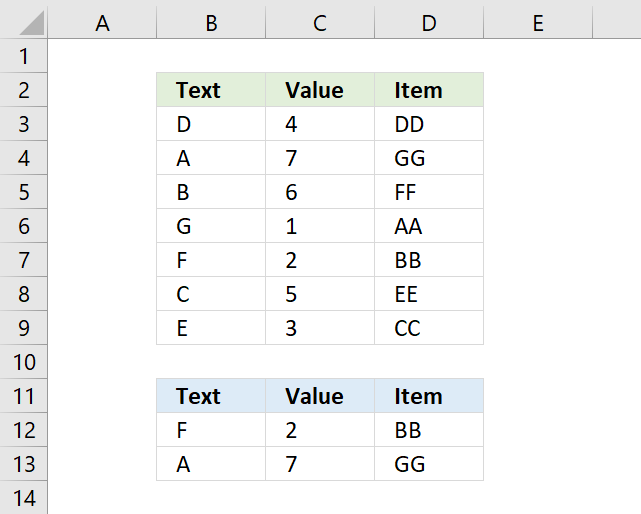This article demonstrates formulas that match two conditions in a column each and return another value on the same row from a column you choose.

Section 3 shows how to do a case-sensitive match using two conditions, and section 4 shows how to do a partial match using two conditions.

## 1. Match two columns and return another value on the same row

###The array formula in cell D12 matches two values in two columns each and returns a value on the same row. First condition is specified in cell B12 and the second condition in cell C12.

The formula returns a value from cell range D3:D9 if both conditions are met on the same row. The example above returns cell value "NN" from cell D7.

Cell value "F" and "2" are found in cells B7 and C7, the corresponding value from cell D7 is returned to cell D12.

Formula in cell D12:

=INDEX(\$D\$3:\$D\$9, MATCH(1, COUNTIFS(B12, \$B\$3:\$B\$9, C12,\$C\$3:\$C\$9) ,0))

If you are looking for a way to compare two columns for differences or compare two columns for same values, please press with left mouse button on links.

You are not limited to formulas, conditional formatting allows you to compare two columns and highlight matches or compare two columns and highlight differences.

### 1.1 How to enter an array formula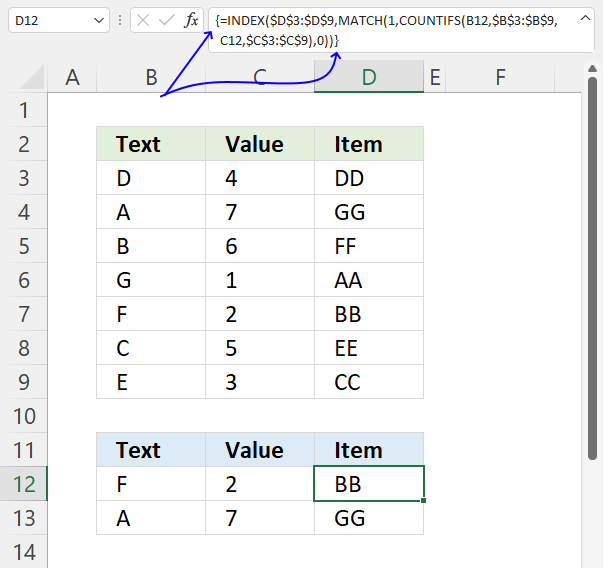To enter an array formula, type the formula in a cell then press and hold CTRL + SHIFT simultaneously, now press Enter once. Release all keys.

The formula bar now shows the formula enclosed with curly brackets telling you that you entered the formula successfully. Don't enter the curly brackets yourself.

Note that Excel 365 users can enter the formula as a regular formula.

### 1.2 Explaining formula

#### Step 1 - COUNT cells based on criteria

The COUNTIFS function counts rows where both values match, however, it returns an array that corresponds to the number of rows in cell range B3:D9.

COUNTIFS(B12, \$B\$3:\$B\$9, C12,\$C\$3:\$C\$9)

returns {0; 0; 0; 0; 1; 0; 0} , shown in column F in picture below.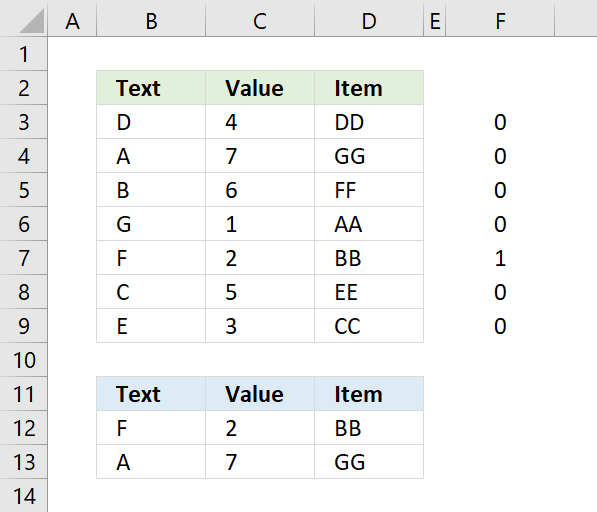Both values match in row 7.

#### Step 2 - Calculate the relative position

The MATCH function returns a number representing the relative position of an item in an array or cell reference that matches a specified value in a specific order.

MATCH(lookup_value, lookup_array, [match_type])

MATCH(1, COUNTIFS(B12, \$B\$3:\$B\$9, C12,\$C\$3:\$C\$9) ,0)

The MATCH function identifies the relative position of the matching values.

MATCH(1, {0;0;0;0;1;0;0} ,0)

and returns 5, 1 is the fifth value in the array.

#### Step 3 - Get value

The INDEX function returns a value from a given cell range based on a row and column number (optional).

INDEX(array, [row_num], [column_num], [area_num])

INDEX(\$D\$3:\$D\$9, MATCH(1, COUNTIFS(B12, \$B\$3:\$B\$9, C12,\$C\$3:\$C\$9) ,0))

The INDEX function returns the corresponding value from column D.

INDEX(\$D\$3:\$D\$9, 5)

and returns BB in cell D12.

## 2. Match two columns and return another value on the same row - regular formulaThis example is identical to the example above, however, it uses a regular formula mot an array formula.

Formula in cell D12:

=INDEX(\$D\$3:\$D\$9, MATCH(1, INDEX(COUNTIFS(B12, \$B\$3:\$B\$9, C12, \$C\$3:\$C\$9), ), 0))

The above formula is a regular formula, it is slightly larger than the first formula demonstrated above in section 1.

## 3. Match two columns and return another value on the same row - case sensitive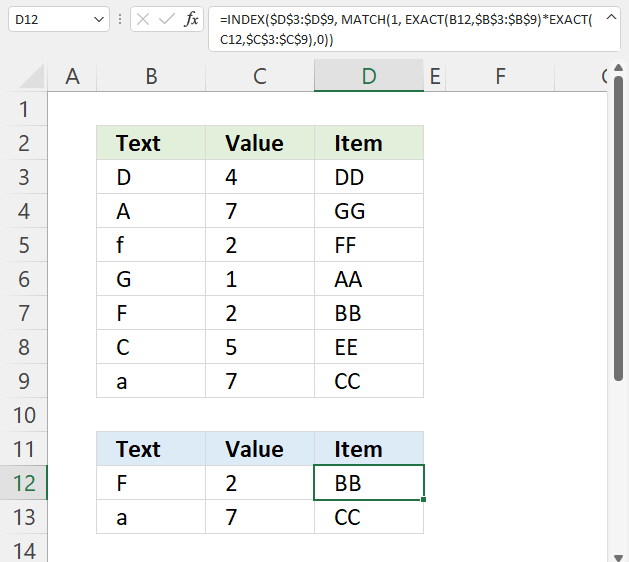Formula in cell D12:

=INDEX(\$D\$3:\$D\$9, MATCH(1, EXACT(B12,\$B\$3:\$B\$9)*EXACT(C12,\$C\$3:\$C\$9),0))

### 3.1 Explaining formula in cell D12

#### Step 1 - Identify values equal to value in cell B12 considering upper and lower case letters

The EXACT function lets you compare values also considering upper and lower cases.

EXACT(text1text2)

EXACT(B12,\$B\$3:\$B\$9)

becomes

EXACT("F",{"D";"A";"f";"G";"F";"C";"a"})

and returns

{FALSE; FALSE; FALSE; FALSE; TRUE; FALSE; FALSE}.

#### Step 2 - Identify values equal to value in cell C12 considering upper and lower case letters

EXACT(C12,\$C\$3:\$C\$9)

becomes

EXACT(2, {4; 7; 2; 1; 2; 5; 7})

and returns

{FALSE; FALSE; TRUE; FALSE; TRUE; FALSE; FALSE}.

#### Step 3 - Multiply arrays AND logic

To apply AND logic we must multiply the arrays using the asterisk character.

EXACT(B12,\$B\$3:\$B\$9)*EXACT(C12,\$C\$3:\$C\$9)

becomes

{FALSE; FALSE; FALSE; FALSE; TRUE; FALSE; FALSE} * {FALSE; FALSE; TRUE; FALSE; TRUE; FALSE; FALSE}

and returns {0; 0; 0; 0; 1; 0; 0}.

#### Step 4 - Find position

The MATCH function returns the relative position of an item in an array or cell reference that matches a specified value in a specific order.

MATCH(lookup_value, lookup_array, [match_type])

MATCH(1, EXACT(B12,\$B\$3:\$B\$9)*EXACT(C12,\$C\$3:\$C\$9),0)

becomes

MATCH(1, {0; 0; 0; 0; 1; 0; 0},0)

and returns 5.

#### Step 5 - Get value based on row number

The INDEX function returns a value from a cell range, you specify which value based on a row and column number.

INDEX(array[row_num][column_num], [area_num])

INDEX(\$D\$3:\$D\$9, MATCH(1, EXACT(B12,\$B\$3:\$B\$9)*EXACT(C12,\$C\$3:\$C\$9),0))

becomes

INDEX(\$D\$3:\$D\$9, 5)

becomes

INDEX({"DD"; "GG"; "FF"; "AA"; "BB"; "EE"; "CC"}, 5)

and returns "BB" in cell D12.

## 4. Match two columns and return another value on the same row - partial match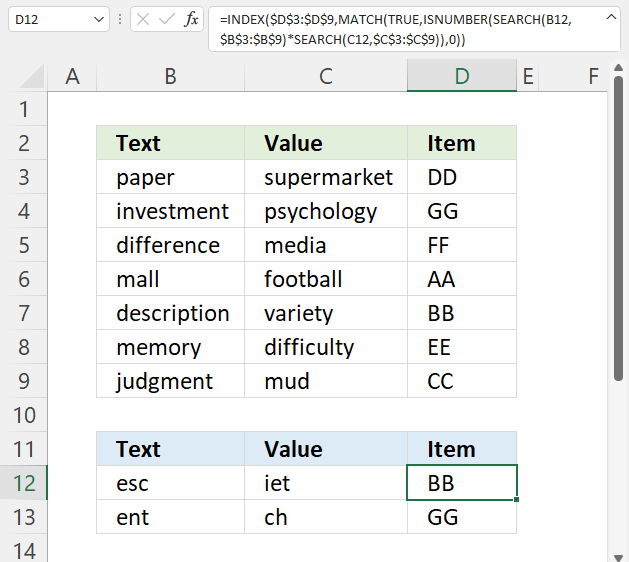Formula in cell D12:

=INDEX(\$D\$3:\$D\$9, MATCH(TRUE, ISNUMBER(SEARCH(B12, \$B\$3:\$B\$9)*SEARCH(C12, \$C\$3:\$C\$9)), 0))

### 4.1 Explaining formula in cell D12

#### Step 1 - Find position of first condition in string

The SEARCH function returns a number representing the position of character at which a specific text string is found reading left to right. The function is not doing a case-sensitive search.

SEARCH(find_text,within_text, [start_num])

SEARCH(B12, \$B\$3:\$B\$9)

becomes

SEARCH("esc",{"paper"; "investment"; "difference"; "mall"; "description"; "memory"; "judgment"})

and returns

{#VALUE!; #VALUE!; #VALUE!; #VALUE!; 2; #VALUE!; #VALUE!}

Notice the #VALUE! error, this is what you get if the string is not found.

#### Step 2 - Find position of the second condition in string

SEARCH(C12, \$C\$3:\$C\$9)

becomes

SEARCH("iet", {"supermarket"; "psychology"; "media"; "football"; "variety"; "difficulty"; "mud"})

and returns

{#VALUE!; #VALUE!; #VALUE!; #VALUE!; 4; #VALUE!; #VALUE!}.

#### Step 3 - Multiply arrays

Both arrays must return a number in the same position in the array, this can be created using the asterisk sign and multiplying the arrays.

SEARCH(B12, \$B\$3:\$B\$9)*SEARCH(C12, \$C\$3:\$C\$9)

becomes

{#VALUE!; #VALUE!; #VALUE!; #VALUE!; 2; #VALUE!; #VALUE!}* {#VALUE!; #VALUE!; #VALUE!; #VALUE!; 4; #VALUE!; #VALUE!}

and returns

{#VALUE!; #VALUE!; #VALUE!; #VALUE!; 8; #VALUE!; #VALUE!}.

#### Step 4 - Find the numbers in the array

The ISNUMBER function checks if a value is a number, returns TRUE or FALSE.

ISNUMBER(value)

ISNUMBER(SEARCH(B12, \$B\$3:\$B\$9)*SEARCH(C12, \$C\$3:\$C\$9))

becomes

ISNUMBER({#VALUE!; #VALUE!; #VALUE!; #VALUE!; 8; #VALUE!; #VALUE!})

and returns

{FALSE; FALSE; FALSE; FALSE; TRUE; FALSE; FALSE}.

#### Step 5 - Find the position of the first number in the array

The MATCH function returns the relative position of an item in an array or cell reference that matches a specified value in a specific order.

MATCH(lookup_value, lookup_array, [match_type])

MATCH(TRUE, ISNUMBER(SEARCH(B12, \$B\$3:\$B\$9)*SEARCH(C12, \$C\$3:\$C\$9)), 0)

becomes

MATCH(TRUE, {FALSE; FALSE; FALSE; FALSE; TRUE; FALSE; FALSE}, 0)

and returns 5. TRUE is the fifth value in the array.

#### Step 6 - Get value based on position

The INDEX function returns a value from a cell range, you specify which value based on a row and column number.

INDEX(array[row_num][column_num], [area_num])

INDEX(\$D\$3:\$D\$9, MATCH(TRUE, ISNUMBER(SEARCH(B12, \$B\$3:\$B\$9)*SEARCH(C12, \$C\$3:\$C\$9)), 0))

becomes

INDEX(\$D\$3:\$D\$9, 5)

and returns "BB". Value "BB" is the fifth value in D3:D9.